# Convex Set in R^n Problem

• Onezimo Cardoso
In summary, we have shown that if ##x \in \mathbb{R}^n## and ##\overline{x} \in C## are points that satisfy ##|x-\overline{x}|=d(x,C)##, then for all ##y \in C##, we have ##\langle x-\overline{x}, y-\overline{x} \rangle \leq 0##. This is proven by assuming the contrary and showing that it leads to a contradiction. This result is important in the study of convex sets and can be applied in various areas of mathematics and other fields.

## Homework Statement

Let ##C \subset \mathbb{R}^n## a convex set. If ##x \in \mathbb{R}^n## and ##\overline{x} \in C## are points that satisfy ##|x-\overline{x}|=d(x,C)##, proves that ##\langle x-\overline{x},y-\overline{x} \rangle \leq 0## for all ##y \in C##.

## Homework Equations

By definition we have ##d(x,C) = inf \{ |x-z| ; z \in C \}##.
And if ##C## is a convex set, then for any two points ##v,w \in C## we have ##(1-t)v+tw \in C##.

## The Attempt at a Solution

Due the fact that ##|x-\overline{x}|=d(x,C)## , I know that ##|x-\overline{x}|^2 \leq |x-z|^2## for all ##z \in C##.
I tried to sketch a prove by contraction. So I supposed that there is an ##y \in C## that ##\langle x-\overline{x},y-\overline{x} \rangle > 0##.
Then I used the fact that ##C## is a convex set, so ##z=(1-t)y+t\overline{x} \in C##, where ##t \in [0,1]##.
Then I intend to conclude something like ##|x-z|^2 < |x-\overline{x}|^2##, what would be an absurd: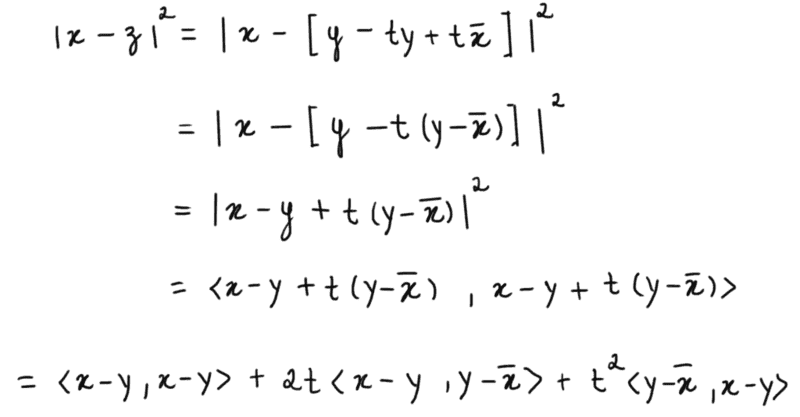But the fact is I came nowhere with my attempt... =(

#### Attachments

If I draw a picture, then the law of cosine and the triangle inequality will come to mind, especially as such an inner product is part of the law of cosine.

Onezimo Cardoso said:

## Homework Statement

Let ##C \subset \mathbb{R}^n## a convex set. If ##x \in \mathbb{R}^n## and ##\overline{x} \in C## are points that satisfy ##|x-\overline{x}|=d(x,C)##, proves that ##\langle x-\overline{x},y-\overline{x} \rangle \leq 0## for all ##y \in C##.

## Homework Equations

By definition we have ##d(x,C) = inf \{ |x-z| ; z \in C \}##.
And if ##C## is a convex set, then for any two points ##v,w \in C## we have ##(1-t)v+tw \in C##.

## The Attempt at a Solution

Due the fact that ##|x-\overline{x}|=d(x,C)## , I know that ##|x-\overline{x}|^2 \leq |x-z|^2## for all ##z \in C##.
I tried to sketch a prove by contraction. So I supposed that there is an ##y \in C## that ##\langle x-\overline{x},y-\overline{x} \rangle > 0##.
Then I used the fact that ##C## is a convex set, so ##z=(1-t)y+t\overline{x} \in C##, where ##t \in [0,1]##.
Then I intend to conclude something like ##|x-z|^2 < |x-\overline{x}|^2##, what would be an absurd:
View attachment 230255

But the fact is I came nowhere with my attempt... =(
I think you are close here. I suggest writing the convexity condition as ##z=(1-t)\overline{x}+ty \in C##, that is, swapping ##t## and ##(1-t)##. This gives you points close to ##\overline{x}## when ##t## is small, and that is where you are likely to find a point ##z## that violates the condition ##|x-\overline{x}|=inf \{ |x-z| ; z \in C \}##. Then, if you work through your derivation with this change, you get ##\langle x-\overline{x},y-\overline{x} \rangle## showing up explicitly and you can find a condition on ##t## that gives you what you need.

•Onezimo Cardoso
Onezimo Cardoso said:

## Homework Statement

Let ##C \subset \mathbb{R}^n## a convex set. If ##x \in \mathbb{R}^n## and ##\overline{x} \in C## are points that satisfy ##|x-\overline{x}|=d(x,C)##, proves that ##\langle x-\overline{x},y-\overline{x} \rangle \leq 0## for all ##y \in C##.

## Homework Equations

By definition we have ##d(x,C) = inf \{ |x-z| ; z \in C \}##.
And if ##C## is a convex set, then for any two points ##v,w \in C## we have ##(1-t)v+tw \in C##.

## The Attempt at a Solution

Due the fact that ##|x-\overline{x}|=d(x,C)## , I know that ##|x-\overline{x}|^2 \leq |x-z|^2## for all ##z \in C##.
I tried to sketch a prove by contraction. So I supposed that there is an ##y \in C## that ##\langle x-\overline{x},y-\overline{x} \rangle > 0##.
Then I used the fact that ##C## is a convex set, so ##z=(1-t)y+t\overline{x} \in C##, where ##t \in [0,1]##.
Then I intend to conclude something like ##|x-z|^2 < |x-\overline{x}|^2##, what would be an absurd:
View attachment 230255

But the fact is I came nowhere with my attempt... =(

What theorems about convex sets have already been proved in your course and so are available for you to use? For example, have you seen the "separating hyperplane" theorem?

Finally I done this question!
Thanks for all the help. I could do it following the tip tnich gave to me.
Soon I'll organize the solution and I'll post here as well.

As I promisse, follow a solution for this question.

Let us suppose, by contraction, there is ##y \in C## such that ##\langle x-\overline{x}, y - \overline{x} \rangle > 0##.

Let ##z=ty+(1-t)\overline{x}##, where ##t \in (0,1)##.

By hypothesis, ##C## is convex. Therefore, ##z \in C##.

We have: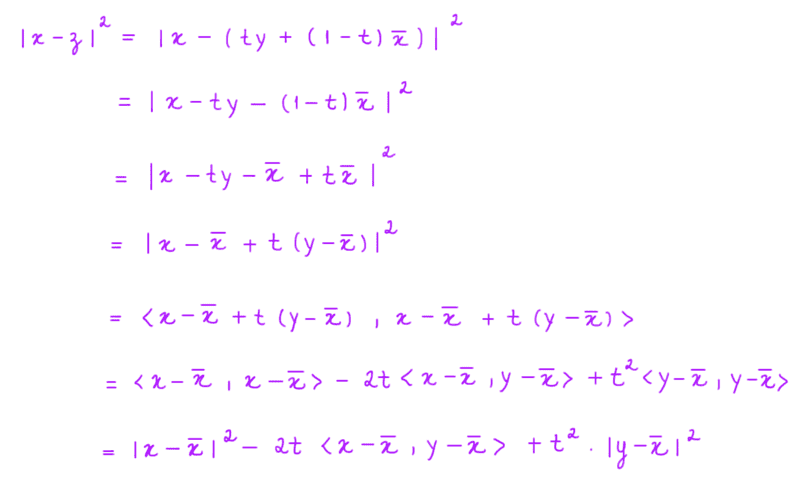Then,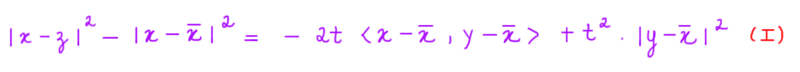By the other hand, we have: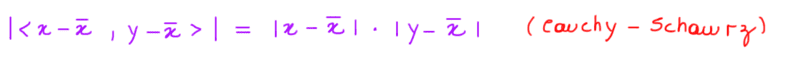By hypothesis, ##\langle x-\overline{x} , y - \overline{x} \rangle > 0##. Therefore, ##| \langle x-\overline{x} , y - \overline{x} \rangle | = \langle x-\overline{x} , y - \overline{x} \rangle##. Then we have: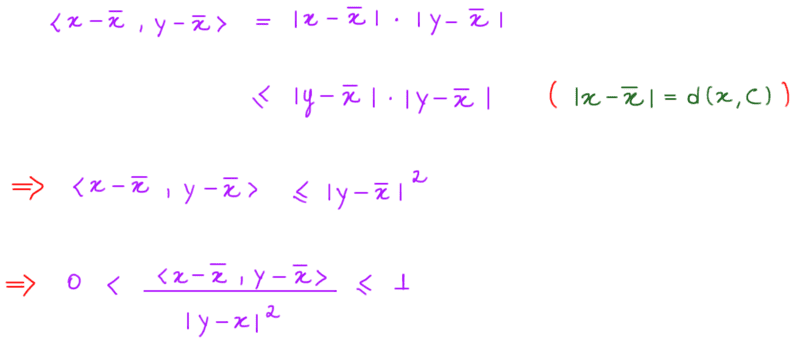Thus,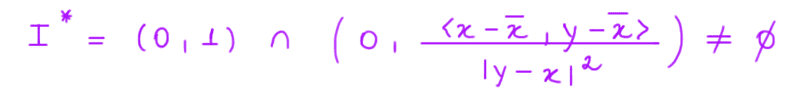Taking ##t \in I^{*}##, we have: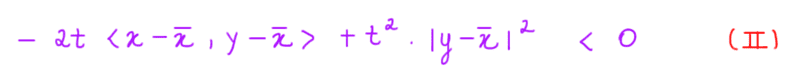Applying ##(II)## in ##(I)##, we have: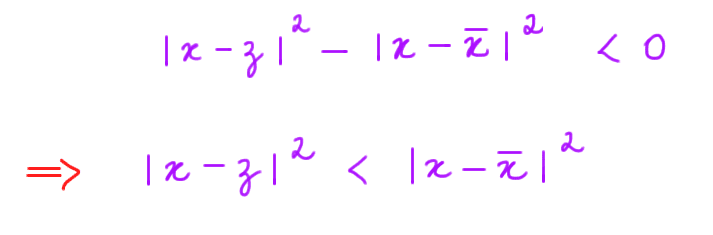Absurd!

Therefore,#### Attachments

•tnich
Another pretty good exercise it to use the exercise above to solve the following one:

Exercise: Let ##C \subset \mathbb{R}^n## be a convex and closed set. Let ##f : \mathbb{R}^n \to C## be a function defined as ##f(x)=\overline{x}##, where ##\overline{x}## is the unique point of ##C## such that ##|x-\overline{x}|=d(x,C)##. Prove that ##|f(x)-f(y)|\leq |x-y|## for any ##x,y \in \mathbb{R}^n##, i.e., ##f## is uniformly continuous.

I could solve this one as well. I'll let it as an exercise, but if someone tries to solve it and cannot find a way I can post my solution here. Thanks guys!

Onezimo Cardoso said:
Another pretty good exercise it to use the exercise above to solve the following one:

Exercise: Let ##C \subset \mathbb{R}^n## be a convex and closed set. Let ##f : \mathbb{R}^n \to C## be a function defined as ##f(x)=\overline{x}##, where ##\overline{x}## is the unique point of ##C## such that ##|x-\overline{x}|=d(x,C)##. Prove that ##|f(x)-f(y)|\leq |x-y|## for any ##x,y \in \mathbb{R}^n##, i.e., ##f## is uniformly continuous.

I could solve this one as well. I'll let it as an exercise, but if someone tries to solve it and cannot find a way I can post my solution here. Thanks guys!
Looks good except for some minor errors (Cauchy - Schwartz inequality would require a ##\leq## sign, ##|y-\overline x|^2## in the denominator). But there are a couple of major ones as well.
1) you have only completed the proof for ##x \notin C##. When ##x\in C##, ##\langle x-\overline{x},y-\overline{x} \rangle = 0## and the chain of inequalities fails. Fortunately, it is easy to prove this special case.
2) Since ##\overline x \in C##, ##y## can be as close to ##\overline x## as you like, meaning that ##|x- \overline x| \leq |y - \overline x|## is not true in general. The proof is still salvageable, though.

Last edited:
tnich said:
Looks good except for some minor errors (Cauchy - Schwartz inequality would require a ##\leq## sign, ##|y-\overline x|^2## in the denominator). But there are a couple of major ones as well.
1) you have only completed the proof for ##x \notin C##. When ##x\in C##, ##\langle x-\overline{x},y-\overline{x} \rangle = 0## and the chain of inequalities fails. Fortunately, it is easy to prove this special case.
2) Since ##\overline x \in C##, ##y## can be as close to ##\overline x## as you like, meaning that ##|x- \overline x| \leq |y - \overline x|## is not true in general. The proof is still salvageable, though.
Try solving this inequality directly for ##t##:
##-2t\langle x-\overline{x} , y - \overline{x} \rangle + t^2|y-\overline x|^2 <0##